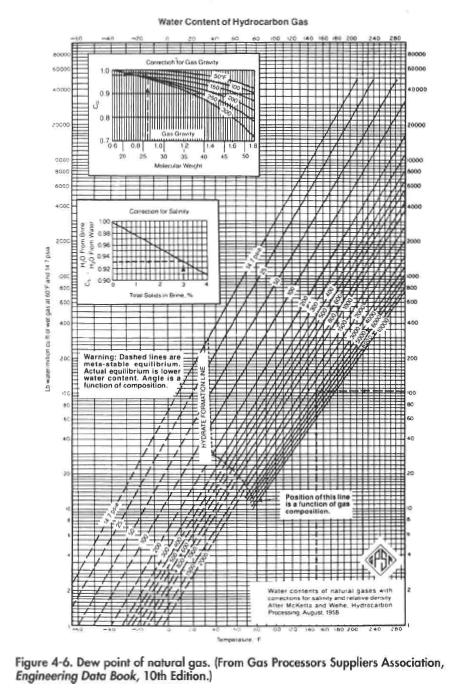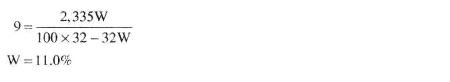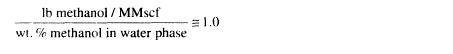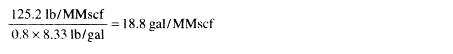﻿ Thermodynamic Inhibitor #2 | Oil & Gas Process Engineering

Thermodynamic Inhibitor #2

First the amount of water that will be condensed will be determined from Figure 4-6, assuming the gas is saturated at reservoir conditions.Water content (8,000 psig and 224°F)= 230 Ib/MMscf
Water content (4,000 psig and 65°F) = JK) Ib/MMscf
Water condensed = 220 Ib/MMscf

From. Table 4-1 the hydrate temperature is 74°F. The required dewpoint depression then will be 74-65 = 9°F.
The concentration of methanol required in the liquid phase from Equation 4-3 is:Desired methanol in liquid phase = 0.11 x 220 Ib/MMscf = 24.2 lb/MMscf

From Figure 4-9 the methanol that will flash to the vapor at 4,000 psig
and 65°F is:Methanol in vapor state = 1.0 x 11.0 = 11.0 Ib/MMscf

Since a barrel of hydrocarbon weighs about 300 Ib, the amount of methanol soluble in the hydrocarbon liquid phase will be:

0.005 x 300 Ib/bbl x 60 bbl/MMscf = 90.0 Ib/MMscf

Thus, the total amount of methanol required:

Water liquid phase                 — 24.21b/MMscf
Vapor phase                             — 11.0 Ib/MMscf
Hydrocarbon liquid phase — 90.0 Ib/MMscf
125.2 Ib/MMscf

Note that the amount of methanol soluble in the condensate is crucial to determining the amount needed. Approximately 125 Ib of methanol must be added so that approximately 25 Ib will be dissolved in the water phase. Since the specific gravity of methanol is 0,8, this is equivalent to:For this case it is impractical to lower the dew point with methanol. A more practical solution would be to separate the condensate first. At 1,000 psia the dew point is 68.4°F . Assuming a separator temperature of 75°F the amount of methanol needed to lower the dew point of gas to 65°F is 9.7 Ib/MMscf. Using a surge factor of 1.4,the required injection rate is only 13.6 Ib/MMscf or 2.0 gal/MMscf.